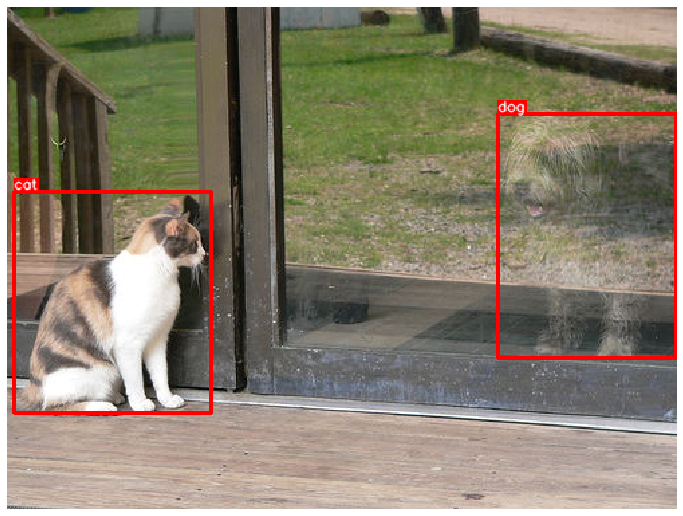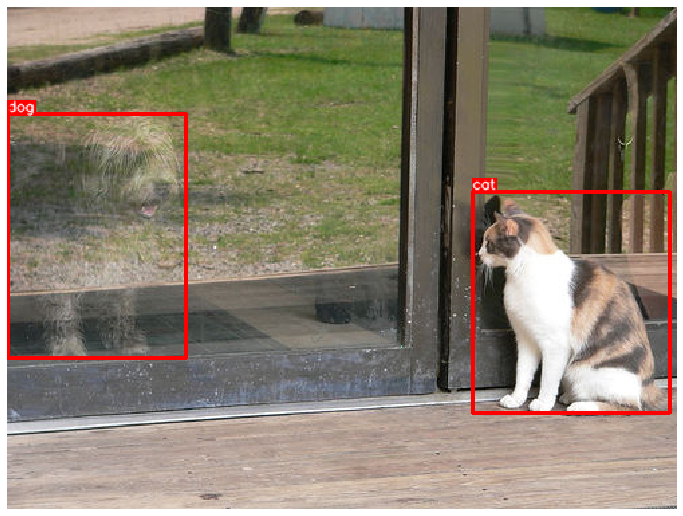# Using Albumentations to augment bounding boxes for object detection tasks¶

### Import the required libraries¶

``````%matplotlib inline
``````
``````import random

import cv2
from matplotlib import pyplot as plt

import albumentations as A
``````

### Define functions to visualize bounding boxes and class labels on an image¶

``````BOX_COLOR = (255, 0, 0) # Red
TEXT_COLOR = (255, 255, 255) # White

def visualize_bbox(img, bbox, class_name, color=BOX_COLOR, thickness=2):
"""Visualizes a single bounding box on the image"""
x_min, y_min, w, h = bbox
x_min, x_max, y_min, y_max = int(x_min), int(x_min + w), int(y_min), int(y_min + h)

cv2.rectangle(img, (x_min, y_min), (x_max, y_max), color=color, thickness=thickness)

((text_width, text_height), _) = cv2.getTextSize(class_name, cv2.FONT_HERSHEY_SIMPLEX, 0.35, 1)
cv2.rectangle(img, (x_min, y_min - int(1.3 * text_height)), (x_min + text_width, y_min), BOX_COLOR, -1)
cv2.putText(
img,
text=class_name,
org=(x_min, y_min - int(0.3 * text_height)),
fontFace=cv2.FONT_HERSHEY_SIMPLEX,
fontScale=0.35,
color=TEXT_COLOR,
lineType=cv2.LINE_AA,
)
return img

def visualize(image, bboxes, category_ids, category_id_to_name):
img = image.copy()
for bbox, category_id in zip(bboxes, category_ids):
class_name = category_id_to_name[category_id]
img = visualize_bbox(img, bbox, class_name)
plt.figure(figsize=(12, 12))
plt.axis('off')
plt.imshow(img)
``````

### Get an image and annotations for it¶

For this example we will use an image from the COCO dataset that have two associated boduning boxes. The image is available at http://cocodataset.org/#explore?id=386298

#### Load the image from the disk¶

``````image = cv2.imread('images/000000386298.jpg')
image = cv2.cvtColor(image, cv2.COLOR_BGR2RGB)
``````

#### Define two bounding boxes with coordinates and class labels¶

Coordinates for those bounding boxes are declared using the `coco` format. Each bounding box is described using four values `[x_min, y_min, width, height]`. For the detailed description of different formats for bounding boxes coordinates, please refer to the documentation article about bounding boxes - https://albumentations.ai/docs/getting_started/bounding_boxes_augmentation/.

``````bboxes = [[5.66, 138.95, 147.09, 164.88], [366.7, 80.84, 132.8, 181.84]]
category_ids = [17, 18]

# We will use the mapping from category_id to the class name
# to visualize the class label for the bounding box on the image
category_id_to_name = {17: 'cat', 18: 'dog'}
``````

#### Visuaize the original image with bounding boxes¶

``````visualize(image, bboxes, category_ids, category_id_to_name)
``````### Define an augmentation pipeline¶

To make an augmentation pipeline that works with bounding boxes, you need to pass an instance of `BboxParams` to `Compose`. In `BboxParams` you need to specify the format of coordinates for bounding boxes and optionally a few other parameters. For the detailed description of `BboxParams` please refer to the documentation article about bounding boxes - https://albumentations.ai/docs/getting_started/bounding_boxes_augmentation/.

``````transform = A.Compose(
[A.HorizontalFlip(p=0.5)],
bbox_params=A.BboxParams(format='coco', label_fields=['category_ids']),
)
``````

We fix the random seed for visualization purposes, so the augmentation will always produce the same result. In a real computer vision pipeline, you shouldn't fix the random seed before applying a transform to the image because, in that case, the pipeline will always output the same image. The purpose of image augmentation is to use different transformations each time.

``````random.seed(7)
transformed = transform(image=image, bboxes=bboxes, category_ids=category_ids)
visualize(
transformed['image'],
transformed['bboxes'],
transformed['category_ids'],
category_id_to_name,
)
``````### Another example¶

``````transform = A.Compose(
[A.ShiftScaleRotate``````